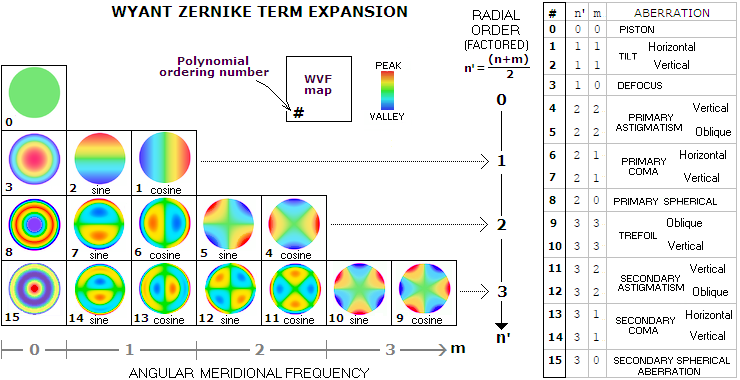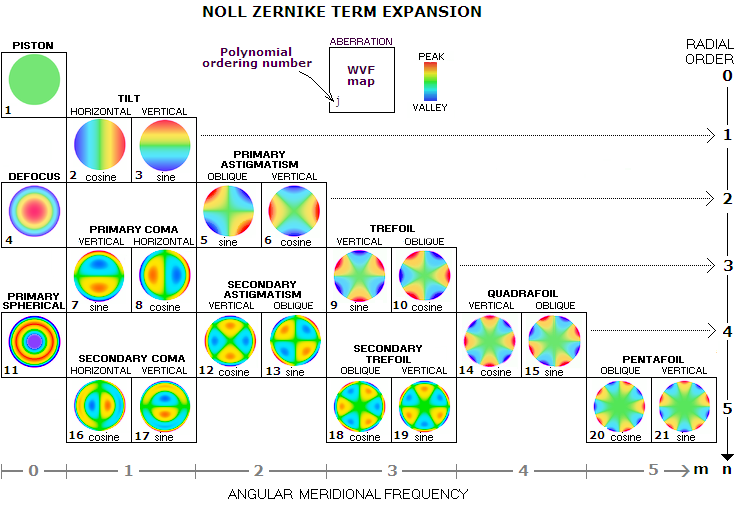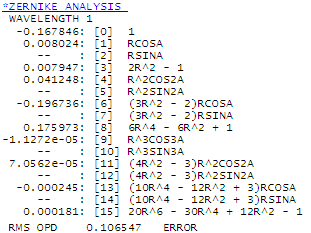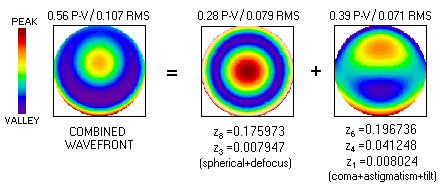telescopeѲptics.net          ▪▪▪▪                                             CONTENTS

# 3.5.3. Zernike expansion schemes

Previous page has a list of the first 15 Zernike terms in Wyant's Zernike expansion scheme, based on their ordering number within the scheme. However, such expansion scheme is arranged with respect to the power integers of Zernike polynomials. A scheme can use power integers n and m as defined here, or somewhat differently, to suit some practical or conceptual aspects of the scheme.

Wyant's Zernike terms expansion can be graphically arranged as shown below for the first 15 terms. Ordering scheme is based on the radial factor n', angular frequency m and θ function, so that terms with lower n' and higher m are ordered first and, for given n' and m, cosine function is ordered before sine function.The scheme has inherent symmetry, in that specific general forms of wavefront deformation become more complex going down vertically, as the higher-order terms develop additional "wrinkles". For instance, for n'=4, the first next term for m=0 (radially symmetrical deformations) is tertiary spherical aberration (#24), for m=1 it is tertiary coma (#23 and #22 for the sine and cosine function, respectively), for m=2 it is tertiary astigmatism (#21 and #20), for m=3 secondary trefoil (#19 and #18) and for m=4 quadrafoil (#17 and #16).

One important difference with respect to other expansion schemes is substitution of the conventional radial order n with (2n'-m) in defining the polynomials, and using n'=(n+m)/2 as a term-defining integer in place of n. In the original paper, n' is denoted by n, same as the conventional radial order integer which is, by definition, the highest power over ρ in the polynomial; since the two are numerically different, the original notation here is changed from n to n' in order to avoid confusion. The polynomial ordering number is used for identifying specific Zernike terms in a single-index notation, as shown in the table at right.

Another Zernike ordering scheme used in the telescope optics domain (e.g. ZEMAX standard Zernike coefficients), based on Noll's concept, is shown below for the first 21 terms. Here, the ordering number j is determined by ordering polynomial with lower radial order first, and for given radial order with odd number for sine function and even number for the cosine.Noll's scheme also has vertical expansion symmetry similar to Wyant's, but since it directly uses radial order n, it unfolds somewhat differently. So for n=6, the term for m=0 is secondary spherical aberration (j=22), for m=2 it is tertiary astigmatism (j=23 and 24 for the sine and cosine function, respectively), for m=4 it is secondary quadrafoil (j=25 and 26) and for m=6 the hexafoil (j=27 and 28).

Yet another relevant Zernike ordering scheme is ANSI (American National Standards Institute) standard single-index scheme (Zernike pyramid) routinely used in ophthalmology for evaluating eye aberrations.EXAMPLE: Zernike wavefront analysis of a 6" f/8 sphere at 0.25° off-axis, for 550nm wavelength. OSLO (which, as mentioned, uses Wyant's expansion scheme for term notation) gives the following non-zero values in the first four decimals (omitting the zero term, piston, which is not an error term with a single aperture): Z1=0.008024 for the tilt, Z3=0.007947 for defocus, Z4=0.041248 for the lower-order (primary) astigmatism, Z6=-0.196736 for lower-order coma, Z8=0.175973 for lower-order spherical, Z13=-0.000245 for higher-order (secondary) coma, and Z15=0.000181 for the secondary spherical (as mentioned, this coefficient is for the balanced form of secondary spherical aberration, or 6th/4th order higher spherical aberration; pure unbalanced secondary spherical will be quantified mostly through the balanced primary spherical coefficient - Z8 - with a smaller residual in Z15, because its wavefront deformation more closely resembles that of the former).

Multiplying the coefficients with the corresponding unit variance RMS values from TABLE 5 (column 6, with the unit variance RMS being 1/N) gives the RMS wavefront error (in units of the wavelength) as Z1 /2 or 0.004012 for the tilt, Z3/3 or 0.004588 for defocus, Z4/6 or 0.016839 for primary astigmatism, Z6/8 or 0.069557 for primary coma, Z8/5 or 0.078698 for primary spherical, Z13/8 or 0.000087 for secondary coma, and Z15/7 or 0.000068 for secondary spherical. The RMS values for lower-order spherical, astigmatism and coma agree with those obtained with standard calculation, given with Eq. 68.2, 70.1 and 71.1, respectively. The combined RMS OPD error on the bottom is a square root of the sum of all individual RMS wavefront (OPD) errors squared.

The secondary spherical RMS value does not correspond to the actual magnitude of this aberration form in a 6" f/8 sphere. As mentioned, the corresponding Zernike coefficient refers to the fully balanced 6th order spherical aberration, which combines pure 6th order spherical, pure 4th order spherical and defocus in order to minimize the aberration (elsewhere in this site it is referred to as balanced 6th/4th order spherical aberration). The P-V error of pure 6th order spherical aberration for mirror surface at paraxial focus is given by Ws6p=(K+1)2d6/8R5, so its magnitude in a 6" f/8 sphere is 0.000000283mm, or 0.000515 in units of 550nm wavelength. Its RMS wavefront error is smaller by a factor of ~0.3, or ~0.000155. When balanced with defocus, the RMS value of the aberration is minimized by a factor of ~0.4, to ~0.000062 (if now combined with a similar amount of 4th order spherical, the RMS reduces to ~0.00001 wave of fully minimized 6th order spherical). Since the above set of Zernike coefficients does not contain one corresponding to balanced 6th order aberration, it is substituted with a similar RMS value of balanced 6th/4th order spherical aberration, Since the latter is a product of similar amounts of 6th and 4th order aberrations, each about six times greater in their RMS magnitude than that of the fully minimized 6th order spherical, the calculation adds as much to the mirror's 4th order component. This, in effect, produces a combination of wavefront forms that corresponds to the mirror's inherent ratio of 4th and 6th order components, resulting in the identical combined wavefront.

That implies that the above conversion relations for spherical aberration will give accurate result for the lower-order only when the higher order term is comparatively negligible, and vice versa, which is usually the case in amateur telescopes (similar limitations may apply to other aberrations as well). Also, Zernike terms for other aberrations need to be comparatively low; if they are not, it indicates asymmetrical wavefront deformation, with the overall RMS value possibly significantly different from that for the spherical aberration component alone.

In addition, the above conversions are valid for clear aperture. In the presence of central obstruction, the RMS error changes somewhat, depending on the relative size of obstruction o and type of aberration, as noted earlier. Both, Zernike aberration term and expansion coefficient should change in proportion. For instance, with 0.25D c. obstruction (o=0.25), all three, the RMS error, ZS and zS, are reduced by a (1-o2)2=0.88 factor.Neglecting higher-order terms, the actual wavefront of a 6" f/8 sphere 0.25° off axis (leftmost) can be decomposed to its two most significant Zernike terms: (1) primary spherical aberration term, with the wavefront map (middle) also including negligible defocus term (it has reversed peak compared to the pattern above because it shows undercorrection), and (2) primary coma term, whose wavefront map is slightly altered by low primary astigmatism and tilt components (right).

Note that the Zernike coefficients given by OSLO were for 550nm wavelength. Their values change in inverse proportion to the wavelength, hence these same values would imply larger error with longer wavelengths, and vice versa. Same as with the standard P-V and RMS wavefront errors, determining specific error level from any given set of Zernike coefficients requires knowledge of the wavelength for which they were calculated.

Another example of interpreting Zernike coefficients is given with wavefront analysis of an off-axis segment mirror.